Robust Sandwich Variance Estimate

For the ith subject,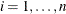, let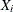,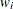, and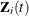be the observed time, weight, and the covariate vector at time t, respectively. Let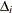be the event indicator and let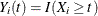. Let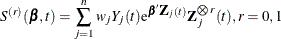Let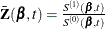. The score residual for the ith subject is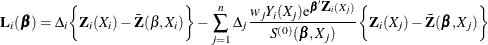For TIES=EFRON, the computation of the score residuals is modified to comply with the Efron partial likelihood. See the section Residuals for more information.

The robust sandwich variance estimate of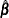derived by Binder (1992), who incorporated weights into the analysis, is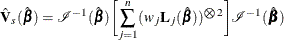where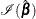is the observed information matrix, and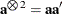. Note that when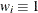,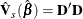where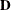is the matrix of DFBETA residuals. This robust variance estimate was proposed by Lin and Wei (1989) and Reid and Crèpeau (1985).